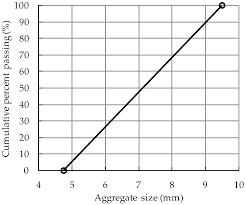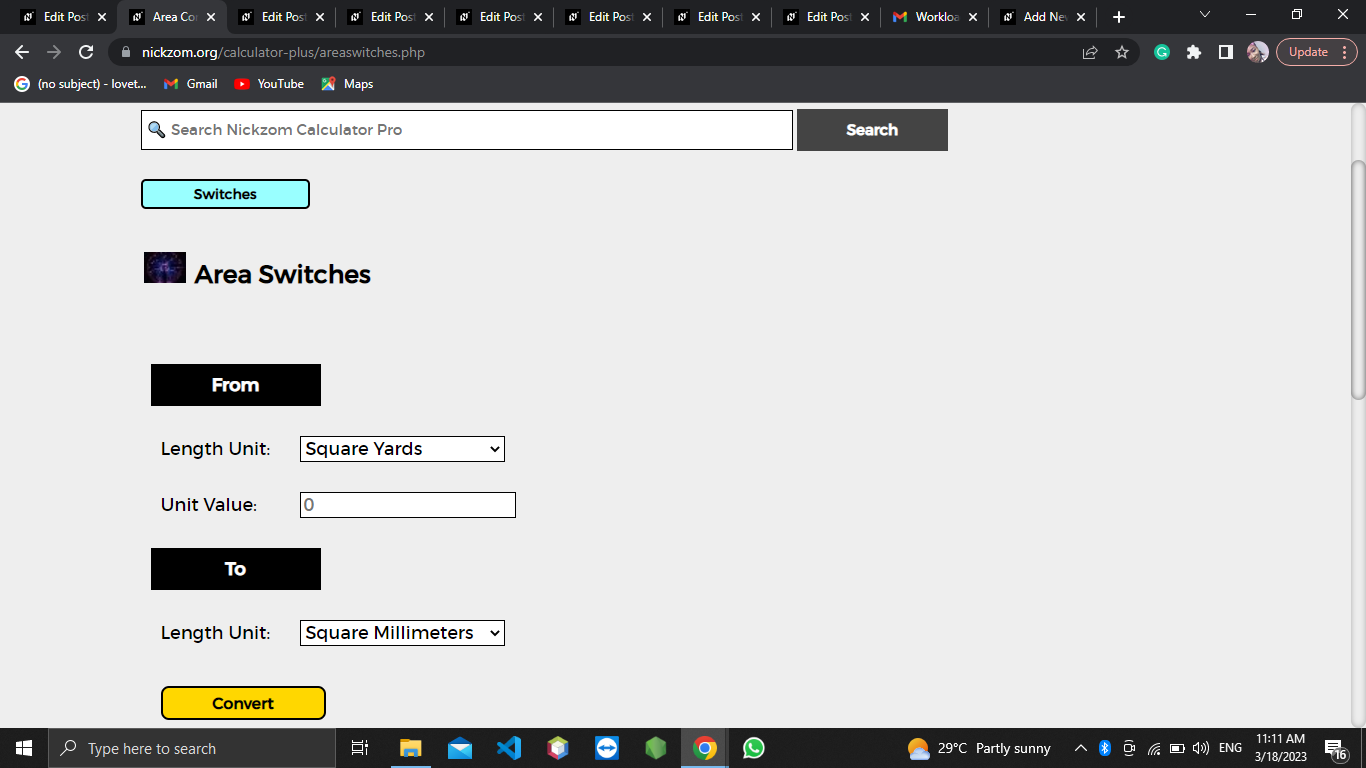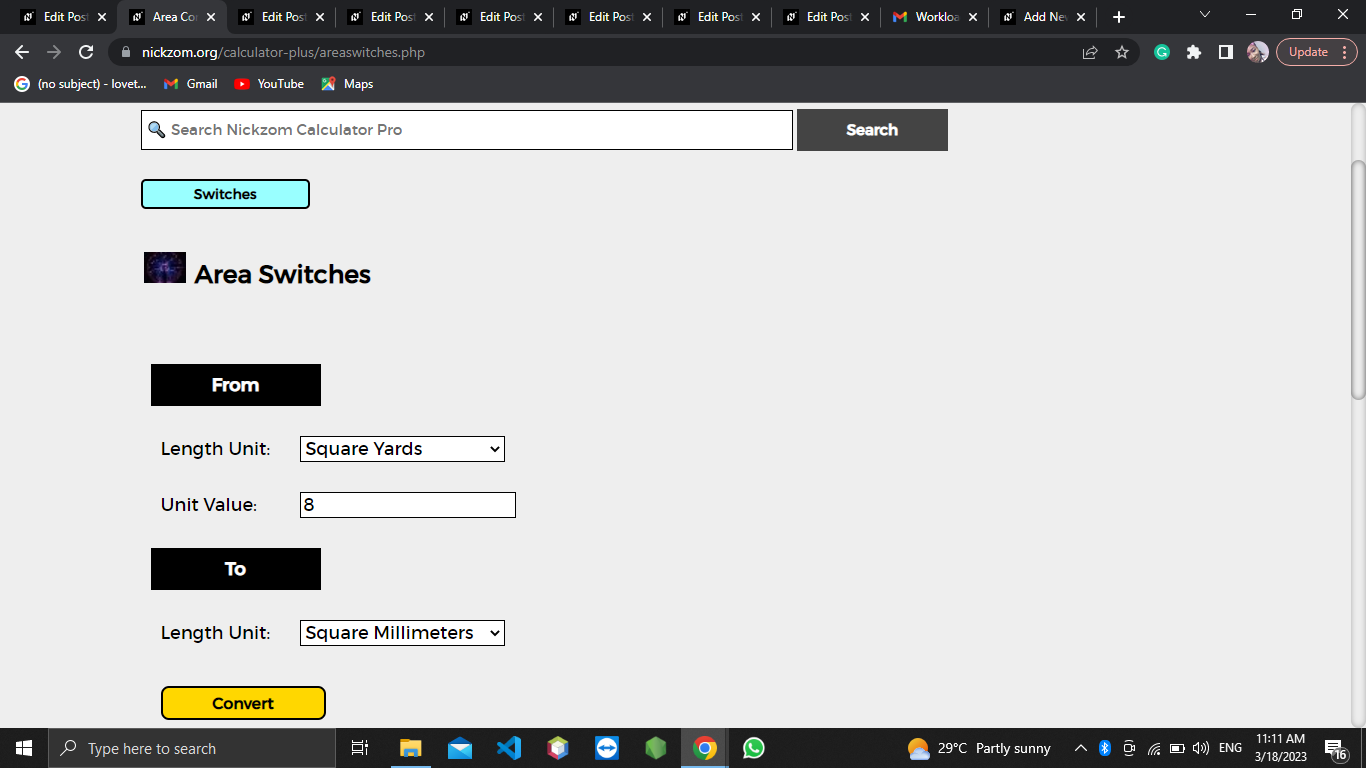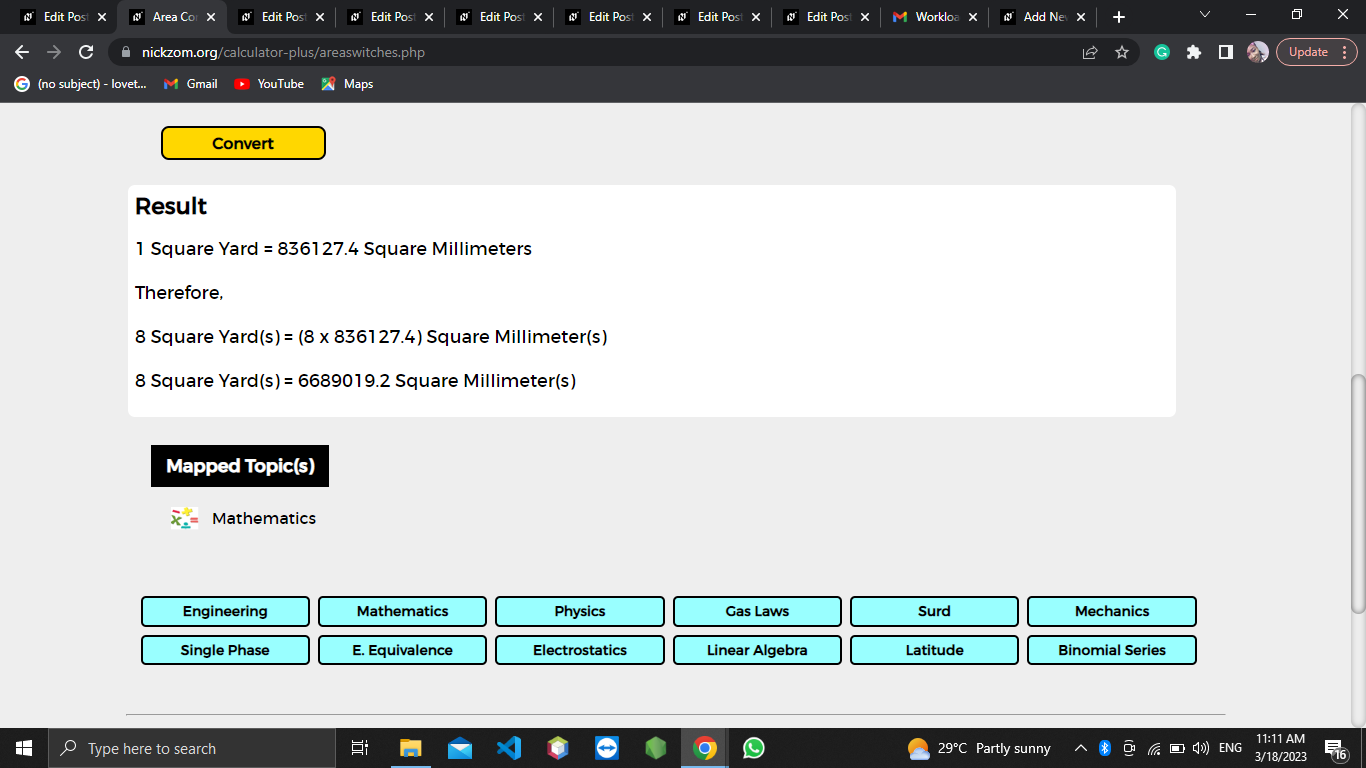# How to Convert Square Yards to Square Millimeters | Area Units

The image below represents square yards to square millimeters.To convert square yards to square millimeters, one essential parameter is needed and this parameter is Unit Value.

The formula for converting square yards to square millimeters:

1 Square Yard = 836127.4 Square Millimeters

Let’s solve an example;
Find the conversion of square yards to square millimeters when the unit value is 8.

1 Square Yard = 836127.4 Square Millimeters

Then,

8 Square Yards = (8 x 836127.4) Square Millimeters
8 Square Yards = 6689019.2 Square Millimeters

Therefore, the square millimeter(s) is 6689019.2.

Nickzom Calculator – The Calculator Encyclopedia is capable of converting square yards to square millimeters.

To get the answer and workings of square yards to square millimeters using the Nickzom Calculator – The Calculator Encyclopedia. First, you need to obtain the app.

You can get this app via any of these means:

You can also try the demo version via https://www.nickzom.org/calculator

Apple (Paid) – https://itunes.apple.com/us/app/nickzom-calculator/id1331162702?mt=8
Once, you have obtained the calculator encyclopedia app, proceed to the Calculator Map, then click on Area Units under Switches.The screenshot below displays the page or activity to enter your value, to get the answer for the conversion of square yards to square millimeters according to the respective parameter which is the Unit Value.Now, enter the value appropriately and accordingly for the parameter as required by the Unit Value is 8.Finally, Click on CalculateAs you can see from the screenshot above, Nickzom Calculator– The Calculator Encyclopedia solves the conversion of square yards to square millimeters and presents the formula, workings, and steps too.